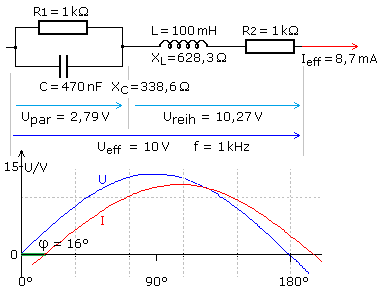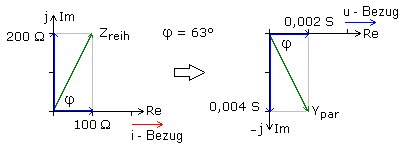# What are examples of a complex circuit

## Impedance calculations in the AC circuit

More extensive resistor networks with only ohmic resistances can be calculated relatively easily using Ohm's law of series and parallel connection. The redrawing and summarizing of ohmic resistance networks applies equally to direct and alternating current technology.

In circuits of alternating current technology with additional capacitive and inductive reactances, voltages and currents show phase shifts with respect to one another. They must be taken into account when calculating the total resistance, the impedance. The exact knowledge and correct application of the vector diagrams provides the key to the calculation approach. Combined LC resistor networks with series and parallel connections of the components must first be converted into their equivalent connections. In the end, you get a pure series or parallel connection of reactive and effective resistances. The impedance of the circuit can be determined with the help of the corresponding phasor diagram.

It is much easier to achieve your goal with the complex calculation. With scientific pocket calculators, you can quickly get the desired result with just a few key combinations. The user must first familiarize himself with the special operation of his computer, as there is no independent standard that the manufacturer must comply with. For the keys R → P, P → R, partly POL and x → y, the necessary conversion formulas are permanently programmed and can be used directly.

### Equivalent circuits

A series connection with active and reactive resistances can be converted into an equivalent parallel connection and vice versa. Both circuits are equivalent if and only if the impedance, i.e. the total resistance and the phase angle, are the same for a certain frequency. In the following example, a series connection is converted into its equivalent parallel connection using the complex calculation. An effective resistance Rreih = 100 Ω and a coil with Lreih = 40 mH form a series connection. For the frequency f = 800 Hz, the component values ​​of the equivalent parallel connection with Rpar and Lpar are sought.The complex impedance of the series connection is calculated from the sum of the two resistance operators. The reference value of each series connection is the current in the direction of the real axis. The operator of the effective resistance lies on the real axis with the pointer length for 100 Ω. The voltage at the inductance leads the current by 90 °. The operator of the inductive reactance pointer length XL = 2 π · f · L = 200 Ω lies on the positive imaginary axis.

The equivalent parallel connection must have the same impedance. With a parallel connection, the voltage is the reference value that lies in the direction of the real axis. The vector diagram of the conductance operators is used. The reciprocal of the admittance Ypar of the parallel circuit is the parallel impedance Zpar and should be equal to the series impedance Zreih of the given circuit. In a step-by-step calculation, the complex denominator becomes real through complex conjugate expansion and the component form for the admittance is obtained. The reciprocal values ​​of the individual components result in the resistance values ​​sought for the equivalent parallel connection.

If the calculator offers the functions mentioned above, the result can be obtained with just a few entries. With R → P the series impedance Zreih is calculated for the series connection and with x → y the phase angle is calculated. The equivalent parallel connection must have the same parallel impedance Zpar and the same phase angle. The reciprocal of the series impedance is thus equal to the admittance of the parallel connection. By changing the reference values, the phase angle has a negative sign for the same value. With the admittance of the P → R function, after entering the negative angular value, the real conductance component G is calculated and with x → y the susceptance component B. It is negative because the conductance operator of the inductance lies on the negative imaginary axis in the parallel connection. The desired resistance values ​​of the components for the equivalent parallel connection follow from the absolute reciprocal values ​​of both components.

The two equivalent circuits are made up of different component values, but have the same electrical properties. It is thus possible to calculate mixed resistance networks in alternating current technology.

### Mixed resistor networks

For the following mixed resistor network, the impedance and the phase angle between voltage and current for a sinusoidal input voltage are calculated using the complex calculation. Both sub-circuits, the RC parallel circuit and the RL series circuit, can be calculated independently of one another. The result is two partial impedances with two phase angles different from 90 °. It is therefore not possible to simply summarize using the Pythagorean theorem.

All resistance values ​​are given or can be calculated from the component values ​​and the frequency. The parallel connection is calculated with the conductance values, their reciprocal resistance values ​​of the components. The resistance values ​​of the components are used for the series connection. Depending on the type of subcircuit, either the complex conductance operators or resistance operators are used for the calculation.In the polar diagram of the Gaussian numerical level, the operator of an effective resistance and its conductance lie on the real axis. The reactance operator and the associated reactance value lie on the imaginary axis. Both are mirrored to each other on the real axis, since the reference value, which is always in the direction of the real axis, changes between current and voltage depending on the type of circuit. A capacitive reactance points down and lies on the negative imaginary axis. The capacitive susceptance points upwards in the direction of the positive imaginary axis. The pointer length corresponds to the calculated resistance value or the reciprocal conductance. The opposite is true for inductive reactance and reactance.The parallel connection is calculated using the complex normal form. The admittance Ypar of the parallel connection, the admittance, is calculated by adding the conductance G of the resistor and the susceptance BC of the capacitor. The impedance Zpar of this parallel connection, its impedance, is the reciprocal of Ypar. The impedance of the series circuit Zreih is calculated in complex notation by adding the effective resistance R2 and the reactance XL of the coil. The total impedance Z of the combined circuit is the addition of the two complex partial impedances.

The pointer of the impedance Z is in the 1st quadrant. The phase angle is positive. The resistor network behaves like a circuit consisting of an effective resistor and a coil. The result in normal form shows the intercepts on the real and imaginary axes. For the exponential form of the impedance, the pointer length is calculated from the square root of the sum of the intercept squares. The phase angle follows from the arctangent of the quotient of the imaginary to the real component.